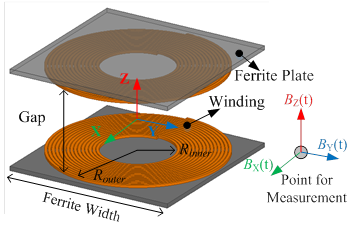LIBRARY

# Synergetic Optimization of Efficiency and Stray Magnetic Field for Planar Coils in Inductive Power Transfer Using Matrix Calculation

Year: 2017 | Author: Ming Lu | Paper: D1.6Fig. 1. Planar coil with ferrite plate in IPT.
When charging the battery of electric vehicles (EVs), inductive power transfer (IPT) uses loosely coupled coils with gaps that are tens of centimeters as a replacement for cables and plugs. Power is transferred from a transmitter to a receiver based on magnetic resonance and near-field magnetic coupling. A planar coil with a spiral winding and magnetic plate, as shown in Fig. 1, is the most popular because of higher coupling across a large air gap and more stable coupling in the presence of misalignment. The coils' efficiency and stray magnetic fields are two important properties for IPT coils. The coils' efficiency is defined as the ratio of active power from the output terminal to the input terminal of the coils. It directly influences the system's effi-ciency. A significant stray magnetic field around the coils is caused by a large gap between the transmitter and receiver.

Efficiency and stray magnetic fields are influenced simultaneously by design of coils' pa-rameters, such as inner radii, outer radii, and distribution of turns. Their synergetic optimization is important. Pareto front of coils' efficiency versus a stray magnetic field is suitable for this kind of multi-objective optimization. Physical parameters are swept in finite-element simulation (FES) to derive the Pareto front. However, the problem of a parametric sweep is that it asks for thou-sands of simulations that are time-consuming especially for 3D simulation.

A faster method using matrix calculations is demonstrated in this paper. Efficiency and magnetic field are calculated with winding vectors, permeance matrices, and current-to-field (I-B) matrices instead of being simulated. Physical parameters of coils including inner radii, outer radii, and distribution of turns are represented with winding vectors. The parametric sweep in simula-tion is replaced by sweeping winding vectors in matrix calculation to derive Pareto fronts for op-timization. Only tens of simulations are required in the optimization procedure using the matrix calculation. Fig. 2 shows the optimization procedure.

Accuracy of the matrix calculation is verified by comparing with experimental measurement for a set of exemplary coils. The maximum difference of the exemplary coils between calculation and measurement is 4% for inductances, 9% for ESRs, and 12.5% for stray magnetic field.Fig. 2. Flowchart of synergetic optimization of coils' efficiency and stray magnetic field for IPT coils.